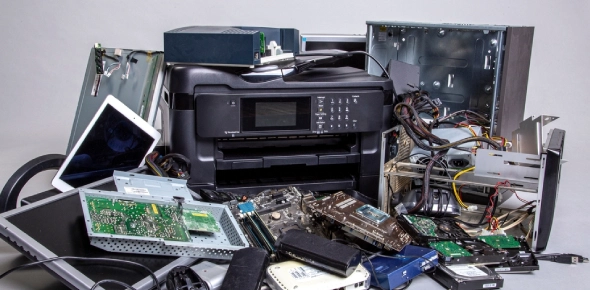# Arduino Quiz #2: Hardware

11 Questions | Attempts: 1054
ShareSettingsA quiz covering some of the Electronics Hardware issues relevant to Arduino users.

• 1.
The circuit show below is used for questions 1-4: A user has connected the circuits above. What is wrong with the circuit connected to pin D2?
• A.

There is no current limiting resistor for the LED.

• B.

Nothing is wrong.

• C.

The LED is inserted backwards.

• D.

Pin D2 may not be used to drive LEDs.

• E.

You should not use an orange wire to connect a red LED.

• 2.
Using the circuits show in Question 1, What is wrong with the circuits connected to pin D3?
• A.

The 5V output of an Arduino Uno is insufficient for a series connection of three LEDs

• B.

The resistor value is too high for this combination of LEDs.

• C.

The resistor value is too low for this combination of LEDs.

• D.

Nothing is wrong with the circuit.

• E.

None of the above

• 3.
Using the circuits show in Question 1: What is wrong with the circuits connected to pin D4?
• A.

The switch needs a pull-down resistor.

• B.

Pin D4 can not be used as a switch input.

• C.

Nothing is wrong with the circuit.

• D.

Switches should have a current limiting resistor.

• E.

None of the above

• 4.
Using the circuits show in Question 1 What is wrong with the circuits connected to pin D5?
• A.

The LED is shorted out.

• B.

The current-limited resistor for the LED has the wrong value.

• C.

Pin D5 should be reserved for Serial

• D.

Nothing is wrong with the circuit.

• E.

None of the above

• 5.
What is wrong with the circuit shown above?  (check all that apply)
• A.

The LED is inserted backwards.

• B.

The Arduino cannot operate from a 9V battery.

• C.

The resistor value is wrong.

• D.

The Arduino is unpowered because the protoboard power rails are not fully connected.

• E.

Pin D8 can not be used to drive an LED.

• 6.
A user has wired up an Arduino Protoshield as shown above. Which of the schematics below match the wiring?
• A.

A

• B.

B

• C.

C

• D.

None of the above

• 7.
The image below is used for several questions. In the circuit shown above, what is the function of components R15 and R16?
• A.

Current Limiting Resistor.

• B.

Pull-up resistor.

• C.

Pull-down resistor.

• D.

None of the above

• 8.
In the image from the question above, what is the function of D8 and D9?
• A.

Kickback prevention diode

• B.

Indicator LED

• C.

Current limiting device

• D.

Light Sensor

• E.

None of the above

• 9.
In the image above, what is the function of R14?
• A.

Current limiting resistor

• B.

Pull-up resistor

• C.

Pull-down resistor

• D.

Light sensor

• E.

None of the above

• 10.
(This is the same image as before; it's repeated for better grouping with the questions) In the image above, what is the function of R17?
• A.

Current limiting resistor

• B.

Pull-up resistor

• C.

Pull-down resistor

• D.

Light Sensor

• E.

None of the above

• 11.
In the image above, what is the function of D10?
• A.

Indicator LED

• B.

Kickback prevention diode

• C.

Pull-up resistor

• D.

Light sensor

• E.

None of the above

## Related TopicsBack to top
×

Wait!
Here's an interesting quiz for you.